# Lagrangian mechanics: central-force-like problem

Homework Statement:
Under the action of gravity, a particle of mass m slides on a conical surface without friction.
The conical surface has the form: ρ=z tan⁡α

a. Find the motion equations using as coordinates θ and r (see picture).
b. Find r_max and r_min if α=30° and initial conditions are:
r(0)=a ; r ̇(0)=0 ; θ ̇^2 (0)=4√3 g/a
c. Find the effective potential and show that circular orbits are possible. Find the particle’s speed in circular orbits.
d. Assuming the particle in circular motion, find the oscillator constant and the period of oscillation for small perturbations of this motion.
Relevant Equations:
Lagrange equations
I copy again the statement here: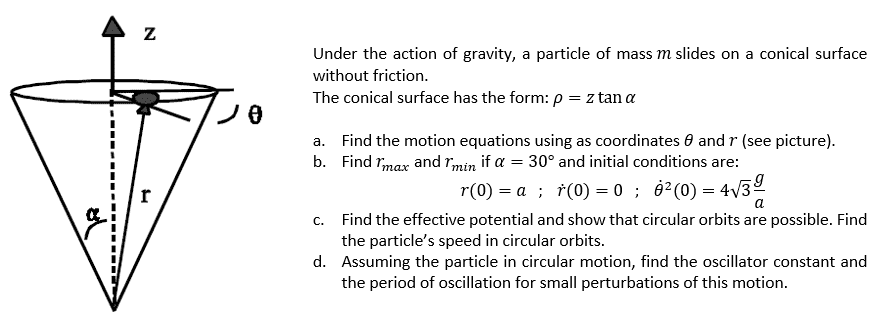So, I think I solved parts a to c but I don't get part d. I couldn't even start it because I don't understand how to set the problem.
I think it refers to some kind of motion like this one in the picture, so I'll have a maximum and a minimum r, and I can get the energy and initial speed of a circular motion from part c, but I don't know how to use them.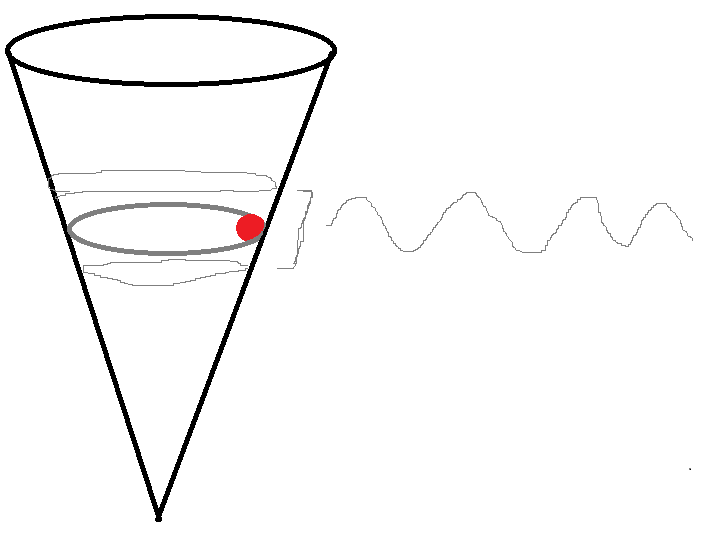I attach my resolution on the other parts.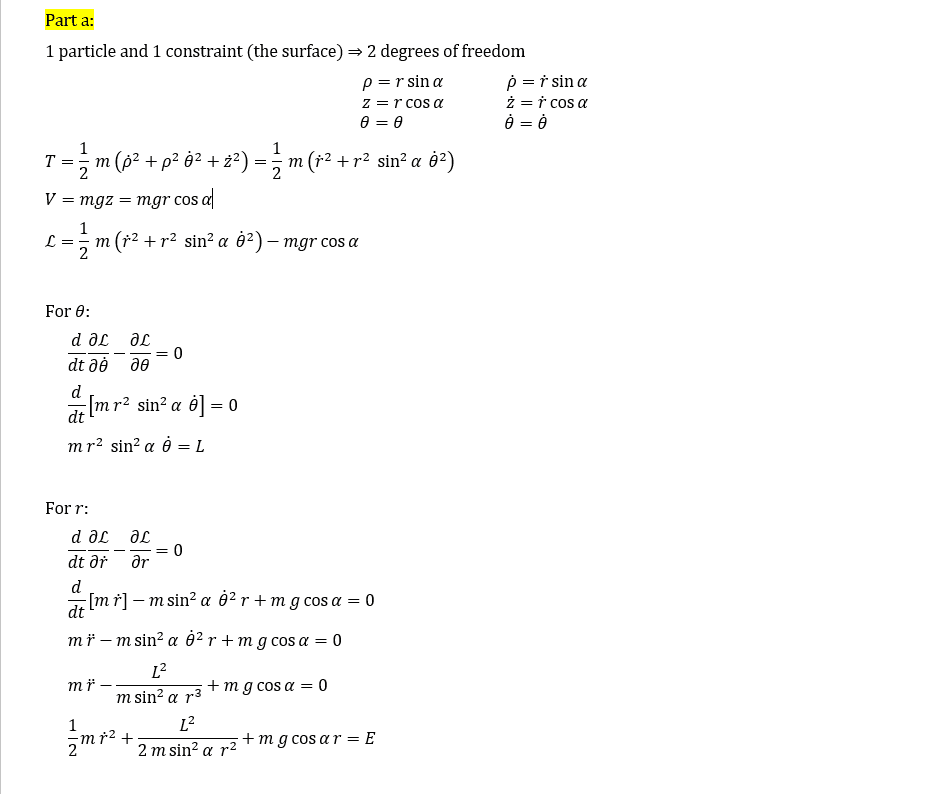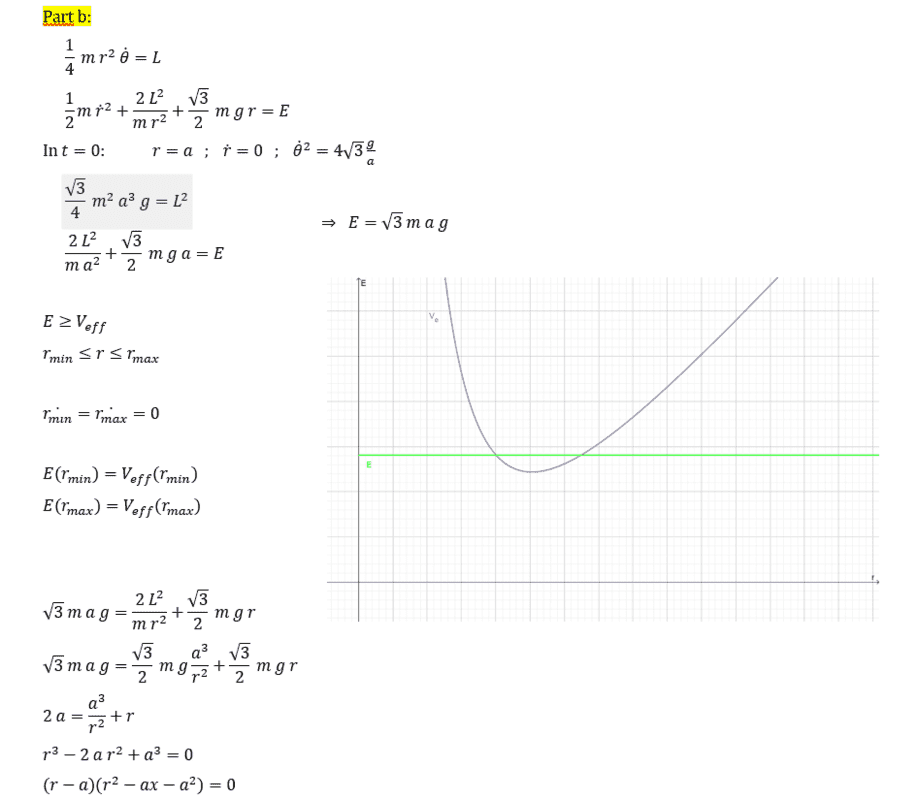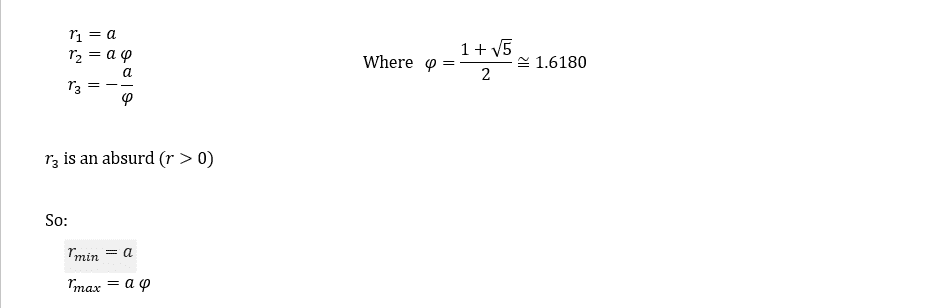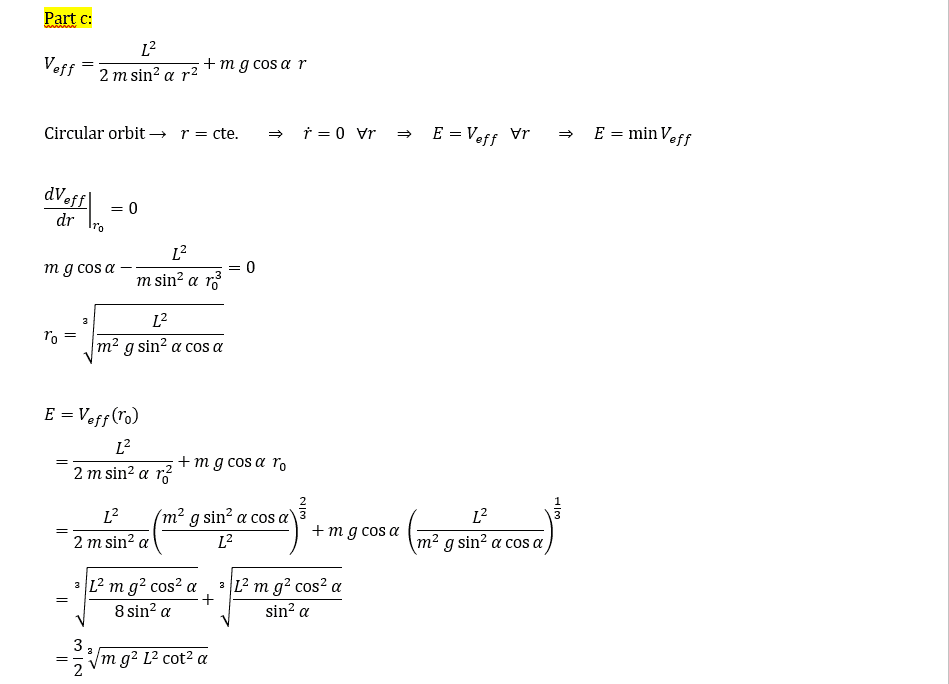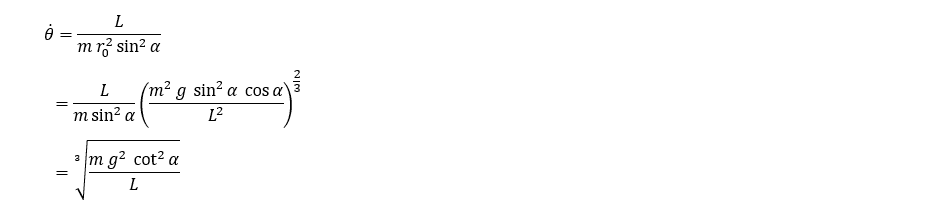#### Attachments

•JD_PM and Delta2

TSny
Homework Helper
Gold Member
You can use ##V_{\rm eff}''(r_0)## to find the "oscillator constant" ##k## and the period of oscillation for small perturbations of the circular motion. See this and also page 2 of this example.

•LuccaP4, JD_PM and Delta2
Many thanks!

Is this valid in every case?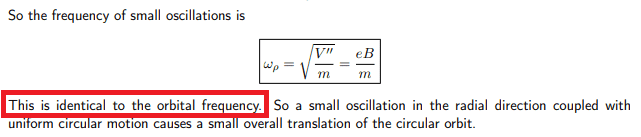In the frecuency ##\omega##, I should evaluate the second derivative in ##r_0##, shouldn't I?

TSny
Homework Helper
Gold Member
Is this valid in every case?
View attachment 263728

In the frecuency ##\omega##, I should evaluate the second derivative in ##r_0##, shouldn't I?
The frequency of small oscillations does not equal the orbital frequency, in general. The example in the link is an interesting exception where they are equal.

Yes, you need to find ##\frac{d^2 V_{\rm eff}(r)}{dr^2} |_{r = r_0}##.

For part (c), I would express ##\dot \theta## in terms of ##r_0##, rather than ##L##. You are also asked to "find the speed". I think this is probably the linear speed of the particle.

For part (d), I would express the "oscillation constant" and the period of the oscillations in terms of the unperturbed radius ##r_0##, rather than in terms of the angular momentum ##L##.

Okay, thanks!